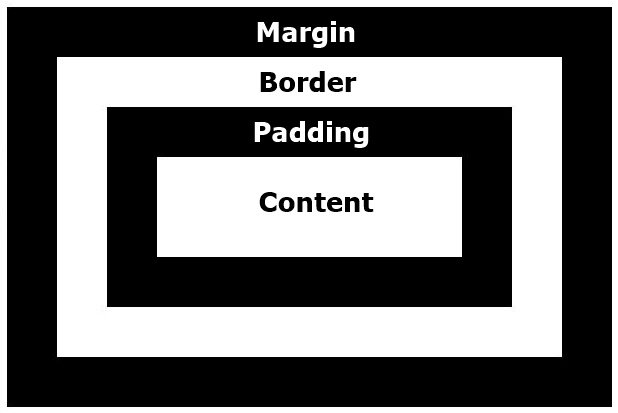CSS Box Model

« Previous Tutorial Next Tutorial »

CSS treats an HTML document as a hierarchical tree of elements, where each element can have zero or more child elements arranged in an ordered way. The topmost element of this tree is called as the root element or the parent element.

These elements display their content at a specific position, which is defined by using CSS properties.

CSS converts the data of HTML elements in the form rectangular boxes, by using a layout model, called the box model, to set the design and layout of HTML documents. This means that the box model determines how HTML`elements are displayed as boxes.

The box model allows placing a border around the elements and also provides space between elements.

Areas of Box Model

Following figure shows the various areas and edges of a box:The table given below describes the area of the box model as shown in the above figure:

Area Description
Content area Displays the content of a document, such as text and images. This is bounded by a rectangle, which is called as the content edge. Note that the content area always appears inside the padding area.
Padding area Specifies the area around the content area. This is bounded by the padding edge. Outside the padding is the border area and the outside boundary of that area is the border edge. Finally, outside the border is the margin area whose outer edge is called the margin edge.
Border area Specifies the area around the padding area. This is bounded by the border edge.
Margin area Specifies the area around the border area. This is bounded by the margin edge.

A box model includes the following types of boxes (describes in the table given here):

Box Type Description
block-level box Represents a box to show a paragraph
line box Represents a box to show a line of text
inline-level box Represents a box to show the words of a line

Note - A block-level box can contain either other block-level boxes, such as a section containing paragraphs or a table containing rows, or line boxes, such as a paragraph containing lines of text.

Note - A line box contains inline-level boxes, such as a line with words in different styles.

Note - An inline-level box can contain either text with other inline-level boxes or a block-level box, such as a small table that is in inline format.

Let's explore various other aspects of the box model in the following sections:

CSS Box Model Example

Here is an example to understand about CSS box model:

<!DOCTYPE html>
<html>
<title>CSS Box Model Example</title>
<style>
div { background-color: white; width: 300px; padding: 15px;
border: 15px solid green; margin: 15px; }
</style>
<body>

<div>
This is codescracker.com, you are watching me in a box which
is created by css box model. Using CSS box model you can make your website
too interactive. In box model, first you have to define the following three things:
<ul>
<li>Margin</li>
<li>Border</li>
</ul>
Then after defining the above three things you can starts writing your
contents inside the box.
</div>

</body>
</html>

Here is the sample output produced by the above CSS box model example code:Note - When you set the width and height properties of an element with CSS, you just set the width and height of the content area. To calculate the full size of an element, you must also add padding, borders and margins.

CSS Online Test

« Previous Tutorial Next Tutorial »